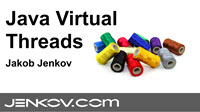Jakob Jenkov Last update: 2014-06-15

The SVG masking feature makes it possible to apply a mask to an SVG shape. The mask determines what parts of the SVG shape that is visible, and with what transparency. You can think of an SVG mask as a more advanced version of a clip path.

Here is a simple mask example:

```<defs>
<rect x="0" y="0" width="100" height="50"
style="stroke:none; fill: #ffffff"/>
</defs>

<rect x="1" y="1" width="100" height="100"
```

This example defines a mask with the ID `mask1`. Inside the `<mask>` element is a `<rect>` element. It is this `<rect>` element which is defines the shape of the mask.

The example also defines a `<rect>` element which uses the mask. The `<rect>` element references the mask ID attribute from inside its `style` attribute, using the `mask` CSS property.

Here is the resulting image:

Notice how the rectangle to be displayed is 100 pixels high, but only the first 50 pixels vertically are visible. That is because the mask rectangle is only 50 pixels high. The rectangle is only visible in the parts covered by the mask rectangle.

The black outlined rectangle is the size of the rectangle it would have had without the mask.

You can use any SVG shape as a mask. Here is an example that uses a circle as mask:

```<svg>
<defs>
<circle cx="25" cy="25" r="25" style="stroke:none; fill: #ffffff"/>
</defs>

<rect x="1" y="1" width="100" height="100"

</svg>
```

Here is the resulting image:

Notice again how the rectangle referencing the mask is only visible where the masking circle is visible.

So far the masking shape (circle or rectangle) had its fill color set to `#ffffff`. The color of the masking shape defines the opacity of the shape that uses the mask. The closer the color of the masking shape is to `#ffffff` (white), the more opaque the shape using the mask will be. The closer the color of the masking shape is to `#000000` (black), the more transparent the shape using the mask will be.

Here is an example where the mask consists of two rectangles with different colors (`#ffffff` and `#66666`). The mask is used on a single rectangle, so you can see how the two different shapes in the mask affects the same shape using the mask.

```<defs>

<rect x="0" y="0"  width="100" height="50"
style="stroke:none; fill: #ffffff"/>

<rect x="0" y="50" width="100" height="50"
style="stroke:none; fill: #666666"/>
</defs>

<text x="10" y="55" style="stroke: none; fill: #000000;">
This text is under the rectangle
</text>

<rect x="1" y="1" width="100" height="100"
```

The example also contains a text under the rectangle, which is visible only through the part of the rectangle where the mask is semi-transparent.

Here is the resulting image:

If you apply a gradient to the shape you use as mask, you can achieve a gradient transparency of the shape the mask is applied to.

Here is an example that defines a gradient, a mask that uses the gradient, a rectangle that uses the mask, and a text under the rectangle, so you can see how its transparency changes with the gradient of the mask:

```<defs>
x1="0%"   y1="0%"
x2="100%" y2="0%"
<stop offset="0%"   stop-color="#ffffff" stop-opacity="1"/>
<stop offset="100%" stop-color="#000000" stop-opacity="1"/>

<rect x="0" y="0"  width="200" height="100"
</defs>

<text x="10" y="55" style="stroke: none; fill: #000000;">
This text is under the rectangle
</text>
<rect x="1" y="1" width="200" height="100"

```

Here is the resulting image:

The gradient mask can be combined with other effects, e.g. a fill pattern. Here is an example where the visible rectangle uses a fill pattern as fill, and a gradient in its mask:

```<defs>

x1="0%"   y1="0%"
x2="100%" y2="0%"
<stop offset="0%"   stop-color="#ffffff" stop-opacity="1"/>
<stop offset="100%" stop-color="#000000" stop-opacity="1"/>

<pattern id="pattern2"
x="10" y="10" width="20" height="20"
patternUnits="userSpaceOnUse" >

<circle cx="10" cy="10" r="10" style="stroke: none; fill: #0000ff; " />

</pattern>

<rect x="0" y="0"  width="200" height="100"
</defs>

<text x="10" y="55" style="stroke: none; fill: #000000;">
This text is under the rectangle
</text>
<rect x="1" y="1" width="200" height="100"
```

Notice how the rectangle to be shown references the fill pattern from its `fill` CSS property, and the mask from its `mask` CSS property.

Here is the resulting image.

## Using Fill Patterns in Masks

You can also use a fill pattern in a mask, thus making the mask the shape of the fill pattern. Here is an example:

```<defs>
<pattern id="pattern1"
x="10" y="10" width="20" height="20"
patternUnits="userSpaceOnUse" >

<circle cx="10" cy="10" r="10" style="stroke: none; fill: #999999" />
</pattern>

<rect x="0" y="0"  width="200" height="100"
style="stroke:none; fill: url(#pattern1)"/>
</defs>

<text x="10" y="55" style="stroke: none; fill: #000000;">
This text is under the rectangle
</text>
<rect x="1" y="1" width="200" height="100"

```

Here is the resulting image. Notice how the rectangle is now semitransparent where the fill pattern draws circles, and fully transparent everywhere else.

 Tweet Jakob Jenkov
Featured Videos